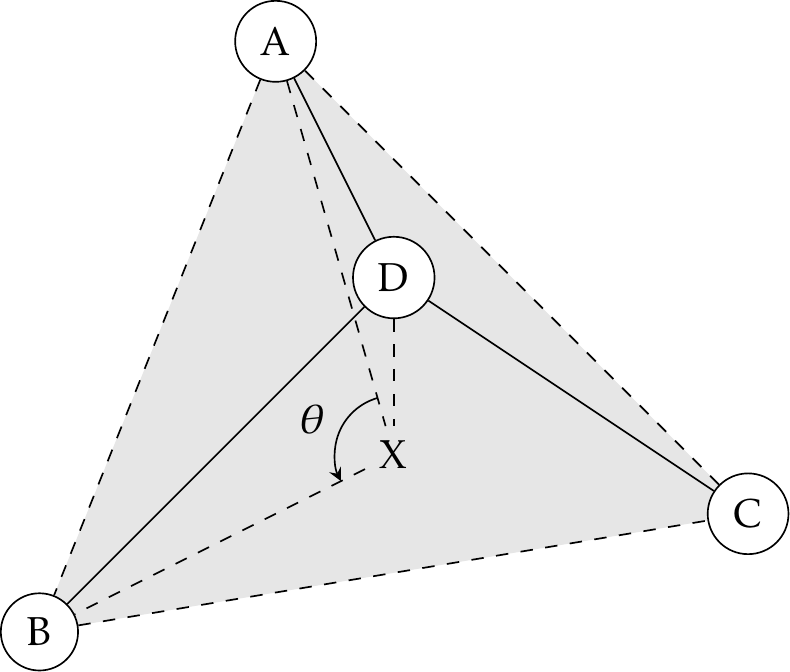# Valence Potential Functions¶

## Bond Stretching¶

Bond term is an empirical function of bond deviating from the ideal bond length, i.e., $$\Delta b = b - b_0$$. To support the AMOEBA force field model, Tinker includes the 3rd and 4th order terms.

$U = k\Delta b^2(1 + k_3\Delta b + k_4\Delta b^2).$

Setting 3rd and 4th order coefficients to zero will give the harmonic functional form.

Note

Different from Hooke’s Law, $$U = k x^2/2$$, Tinker usually drops the coefficient 1/2.

The Morse oscillator is also implemented in Tinker:

$U = D_e [1 - \exp(-a\Delta b)]^2.$

Parameter a is hardwired to 2 by approximation. Following equation $$a = \sqrt{\frac{k}{2 D_e}}$$ and the Tinker convention to include 1/2 in the force constant, De is k/4.

## Angle Bending¶

Similar to bond stretching, angle bending term is also an empirical function of angle deviating from the ideal angle value, i.e., $$\Delta\theta=\theta-\theta_0$$. Terms from cubic to sextic are added to generalize the HARMONIC functional form.

$U = k\Delta\theta^2(1+k_3\Delta\theta+k_4\Delta\theta^2 +k_5\Delta\theta^3+k_6\Delta\theta^4).$

MMFF force field has a special treatment for LINEAR angle, e.g., carbon dioxide. Since the ideal angle should always be $$\pi$$ rad, the deviation can be approximated by

$\Delta\theta=\theta-\pi=2(\frac{\theta}{2}-\frac{\pi}{2})\sim 2\sin(\frac{\theta}{2}-\frac{\pi}{2})=-2\cos\frac{\theta}{2}.$

Only keeping the quadratic term, the angle bending term can be simplified to

$U = 2k(1+\cos\theta).$

The LINEAR angle type is a special case of the SHAPES-style Fourier potential function  with 1-fold periodicity, which is referred to as the FOURIER angle type in Tinker jargon and has the following form

$U = 2k(1+\cos(n\theta-\theta_0)).$

In addition, there is another IN-PLANE angle type for trigonal center atoms. One can project atom D to point X on plane ABC. Instead of angle A-D-B, the ideal and actual angle values are for angle A-X-B (see Fig. 1).Fig. 1 A trigonal center and an in-plane angle.

## Stretch-Bend Coupling¶

The functional forms for bond stretching, angle bending, and stretch-bend coupling are those of the MM3 force field :

$U = (k_1\Delta b_1 + k_2\Delta b_2)\Delta\theta.$

Even though force constants k1 and k2 are implemented as two independent variables in Tinker, they were treated as the same variable in the original literature.

Urey-Bradley potential energy accounts for the 1-3 nonbonded interactions. The cubic and quartic terms are added to the harmonic functional form in Tinker:

$U = k\Delta u^2(1 + k_3\Delta u + k_4\Delta u^2),$

where $$\Delta u$$ is the difference of 1-3 distance deviating from its ideal value. Coefficients k3 and k4 are usually zero.

## Out-of-Plane Bending¶

Tinker has implemented two types of out-of-plane bending angles. In the Wilson-Decius-Cross formulation , the out-of-plane angle $$\chi$$ associated with bond BD in Fig. 1 is the angle between BD and plane ADC, whereas the Allinger formulation uses the angle between BD and plane ABC. Similar to harmonic bond stretching, the following functional form has been implemented in Tinker:

$U = k\chi^2(1 + k_3\chi + k_4\chi^2).$

## Improper Dihedral¶

Commonly used in the CHARMM force fields, this potential function is meant to keep atoms planar. The ideal angle $$\phi_0$$ defined by dihedral D-A-B-C will always be zero degrees. D is the trigonal atom, A-B-C are the peripheral atoms. In the original CHARMM parameter files, the trigonal atom is often listed last as C-B-A-D.

Some of the improper angles are “double counted” in the CHARMM protein parameter set. Since Tinker uses only one improper parameter per site, we have doubled these force constants in the Tinker version of the CHARMM parameters. Symmetric parameters, which are the origin of the “double counted” CHARMM values, are handled in the Tinker package by assigning all symmetric states and using the Tinker force constant divided by the symmetry number.

The harmonic functional form implemented in Tinker is

$U = k(\phi-\phi_0)^2.$

There is no coefficient 1/2 before the force coefficient, which may be different in other software packages.

## Improper Torsion¶

Commonly used in the AMBER force fields, it defines a hypothetical torsional angle for four atoms not successively bonded, e.g., a trigonal center. The functional form is similar to the proper torsion,

$U = \sum_{n=1}^3 V_n[1+\cos(n\phi-\delta_n)],$

where n is the periodicity and $$\delta_n$$ is the corresponding phase shift.

## Torsional Angle¶

The functional form implemented in Tinker is

$U = \sum_{n=1}^6 V_n[1+\cos(n\phi-\delta_n)],$

where n is the periodicity (up to 6) and $$\delta_n$$ is the corresponding phase shift.

## Pi-Orbital Torsional Angle¶

The 2-fold Fourier torsional angle potential to keep a pi-orbital molecular structure (e.g., ethylene) planar.

$U = V[1+\cos(2\phi-\pi)].$

## Torsion-Torsion Coupling¶

The potential energy is extrapolated from a predefined 2-D map with the $$(\phi,\psi)$$ angles.